﻿ 水下球体目标散射声场相位特性
«上一篇文章快速检索 高级检索

 哈尔滨工程大学学报2020, Vol. 41Issue (7): 945-950  DOI: 10.11990/jheu.2019050220

### 引用本文ZHOU Yanling, FAN Jun, WANG Bin. Phase characteristics of acoustic-scattering field for underwater spherical targets[J]. Journal of Harbin Engineering University, 2020, 41(7): 945-950. DOI: 10.11990/jheu.201905022.### 文章历史

Phase characteristics of acoustic-scattering field for underwater spherical targets
ZHOU Yanling , FAN Jun, WANG Bin
Collaborative Innovation Center for Advanced Ship and Deep-Sea Exploration, State Key Laboratory of Ocean Engineering, Shanghai Jiao Tong University, Shanghai 200240, China
Abstract: To study the phase characteristics of acoustic scattering for underwater targets, in this study, we used the Rayleigh normal series solution and the finite element method to calculate the acoustic-scattering field of a rigid sphere, elastic sphere, and spherical shell, and obtained their frequency and spatial distribution characteristics. The results show that the phase of a rigid sphere changes linearly with frequency when ka>2π, the phase jump of an elastic sphere occurs at the resonance frequency, and the phase frequency characteristics of the spherical shell vary with the material and thickness. We conducted backscattering acoustic-scattering experiments on a single-layer spherical shell in a tank and used the all-phase method to extract the acoustic-scattering phase characteristics, which were found to be in good agreement with the simulation results within a frequency range of 20~40 kHz. These findings provide a theoretical basis for the use of the phase characteristics of underwater targets for target recognition and active acoustic stealth.
Keywords: acoustic scattering    finite element method    sphere    spherical shell    echo simulation    all-phase method    phase characteristics

Mitri等介绍了利用传统傅里叶变换提取目标共振散射形态函数相位的方法，但对窗函数的要求很严格。Rayleigh简正级数解的解析方法只能求解少数规则形状目标的声散射相位，为实现复杂目标声散射相位计算，论文提出基于有限元方法的水下目标声散射相位的数值计算模型。以COMSOL Multiphysics软件为计算平台，求解弹性球和单层球壳目标的频域形态函数并进行时域回波仿真，再利用全相位法提取回波相位，得到了和Rayleigh简正级数解相吻合的结果，直接说明了有限元方法是准确、有效的。而已有的研究证明全相位法具有“相位不变性”、无需附加的频率估计和频谱校正措施、精度较高、更优良的抑制频谱泄漏的优势，可应用于回波相位提取。最后开展了单层球壳反向散射实验，利用全相位法获取了其声散射相位特性，与理论预报结果相比吻合较好。

1 水下球体目标声散射相位的计算方法 1.1 基于Rayleigh简正级数解的声散射相位计算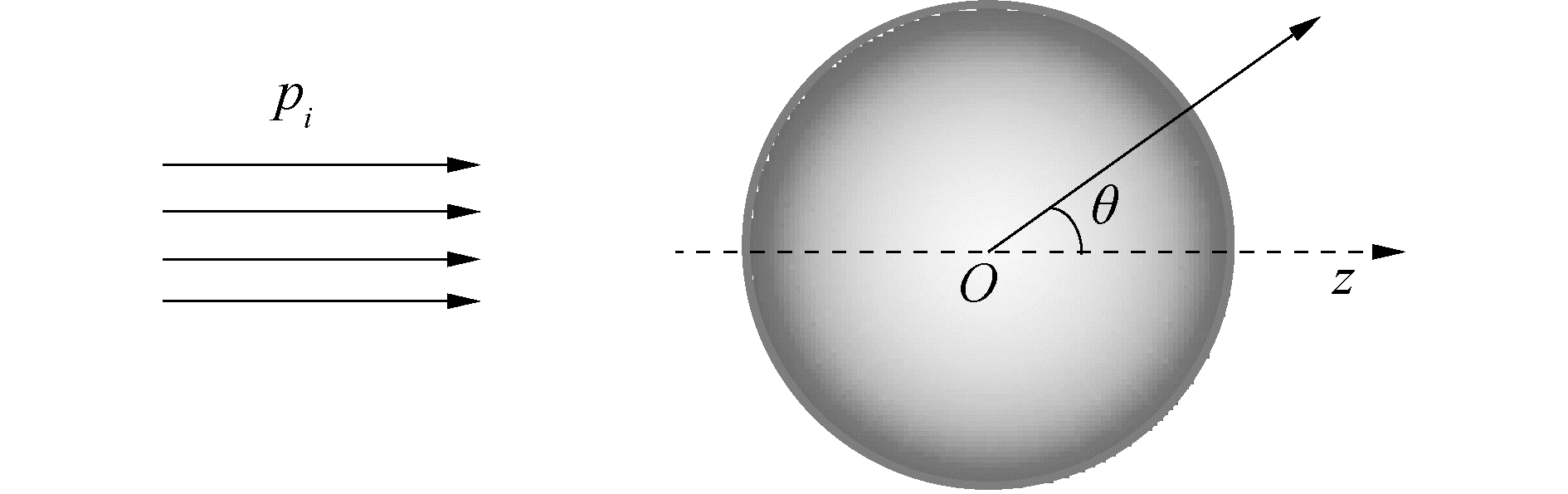Download: 图 1 刚性球的散射 Fig. 1 Scattering of the rigid sphere

 $\begin{array}{l} {p_i}\left( {r, \theta } \right) = \exp \left( {{\rm{i}}kr\cos \theta } \right) = \\ \sum\limits_{n = 0}^\infty {{{\rm{i}}^n}} \left( {2n + 1} \right){{\rm{P}}_n}\left( {\cos \theta } \right){{\rm{j}}_n}\left( {kr} \right) \end{array}$ (1)

 ${p_s}\left( {r, \theta } \right) = \sum\limits_{n = 0}^\infty {{{\rm{i}}^n}} \left( {2n + 1} \right){a_n}{{\rm{P}}_n}\left( {\cos \theta } \right){{\rm{h}}_n}^{\left( 1 \right)}\left( {kr} \right)$ (2)

 $f\left( {x, \theta } \right) = \frac{{2r}}{a}\exp \left( { - {\rm{i}}kr} \right){p_s}\left( {x, \theta } \right)$ (3)Download: 图 2 刚性球表面散射声压相位分布 Fig. 2 Phase distribution of surface scattering sound pressure for the rigid sphere表 1 材料的基本参数 Table 1 Parameters of materials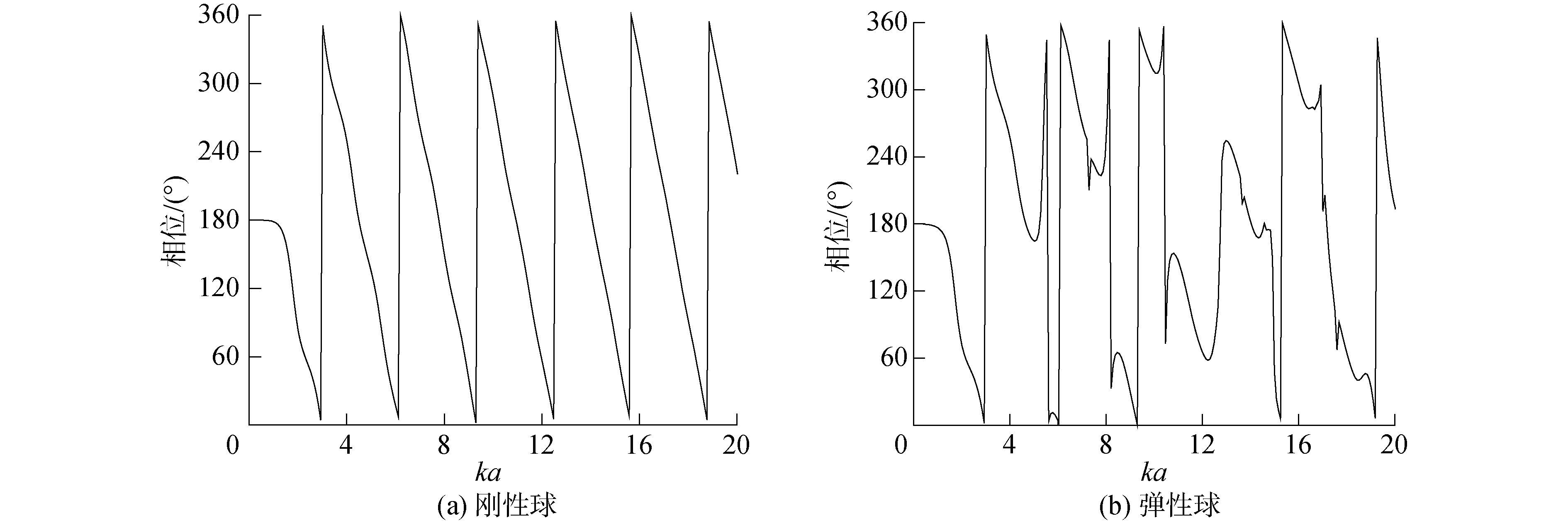Download: 图 3 形态函数相频特性 Fig. 3 Phase-frequency curves of the form functionDownload: 图 4 不同厚度、材料弹性球壳的相频特性 Fig. 4 Phase-frequency curves of the elastic spherical shell with different thickness and materials
1.2 基于有限元声散射相位计算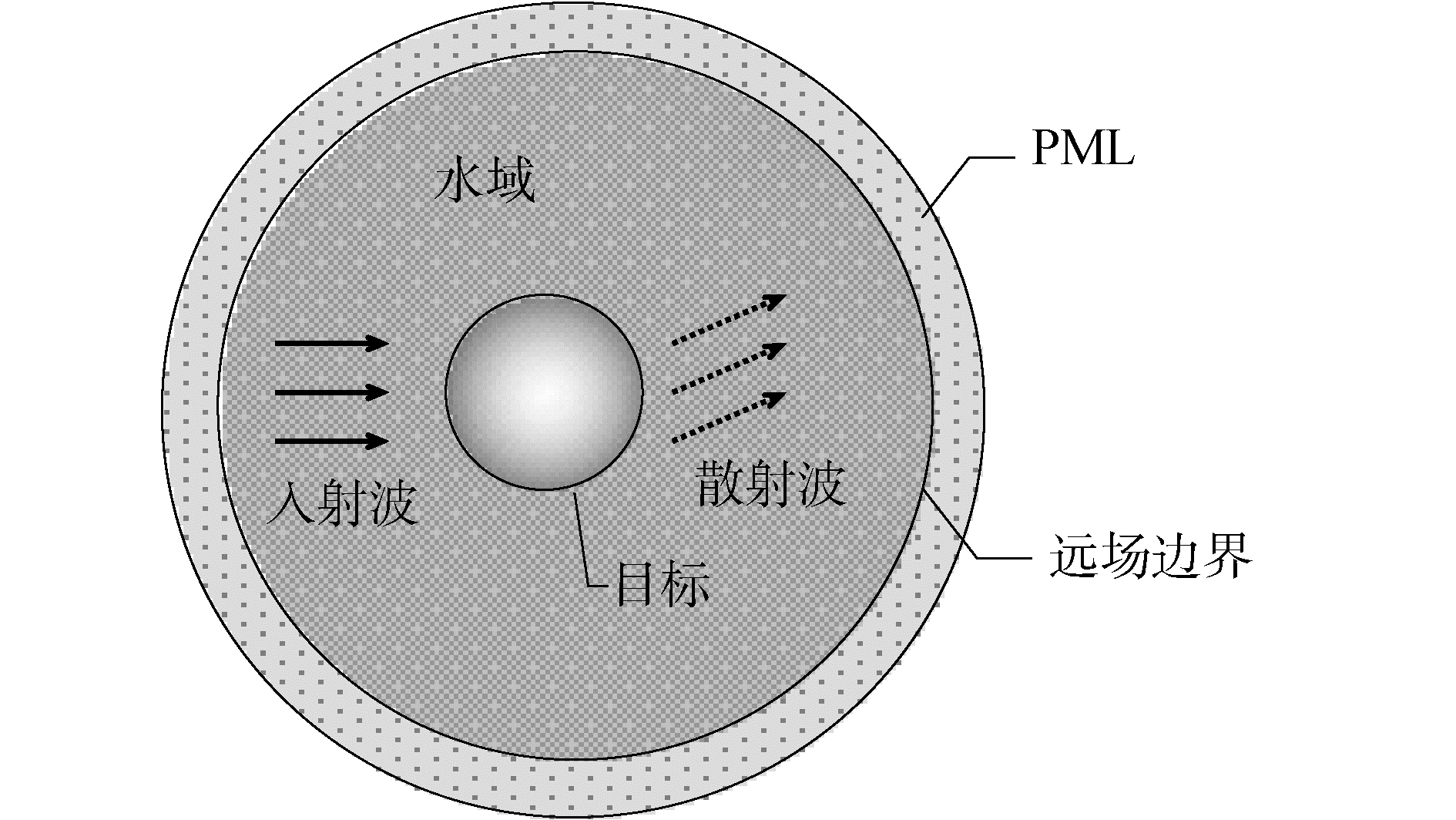Download: 图 5 定义物理场及求解域 Fig. 5 Definition of acoustic scattering field and solving domainDownload: 图 6 有限元和Rayleigh简正级数解对比 Fig. 6 Comparison of the finite element method and Rayleigh normal mode solution
2 散射声场相位的提取方法

 $\begin{array}{l} K\left( k \right) = \frac{1}{N}\sum\limits_{n = 0}^{N - 1} {\left\{ {\exp \left( {{\rm{i}}{\theta _0}} \right)\exp \left( {{\rm{i}}\frac{{2{\rm{ \mathsf{ π} }}nm}}{N}} \right)\exp \left( { - {\rm{i}}\frac{{2{\rm{ \mathsf{ π} }}kn}}{N}} \right)} \right\}} = \\ \frac{1}{N}\frac{{\sin \left[ {{\rm{ \mathsf{ π} }}\left( {m - k} \right)} \right]}}{{\sin \left[ {{\rm{ \mathsf{ π} }}\left( {m - k} \right)/N} \right]}}\exp \left\{ {{\rm{i}}\left[ {{\theta _0} + \left( {1 - \frac{1}{N}} \right){\rm{ \mathsf{ π} }}\left( {m - k} \right)} \right]} \right\} \end{array}$ (4)

x(n)对应的全相位FFT谱为：

 $\begin{array}{l} {X_{ap}}\left( k \right) = \frac{1}{N}\sum\limits_{j = 0}^{N - 1} {{X_j}} \left( k \right)\exp \left( {{\rm{i}}2{\rm{ \mathsf{ π} }}kj/N} \right) = \\ \frac{1}{{{N^2}}}\sum\limits_{j = 0}^{N - 1} {\sum\limits_{n = 0}^{N - 1} {\left\{ {x\left( {n - j} \right)\exp \left( { - {\rm{i}}\frac{{2{\rm{ \mathsf{ π} }}kn}}{N}} \right)\exp \left( {{\rm{i}}\frac{{2{\rm{ \mathsf{ π} }}kj}}{N}} \right)} \right\}} } = \\ \frac{1}{{{N^2}}}\exp \left( {{\rm{i}}{\theta _0}} \right)\frac{{{{\sin }^2}\left[ {{\rm{ \mathsf{ π} }}\left( {m - k} \right)} \right]}}{{{{\sin }^2}\left[ {{\rm{ \mathsf{ π} }}\left( {m - k} \right)/N} \right]}} \end{array}$ (5)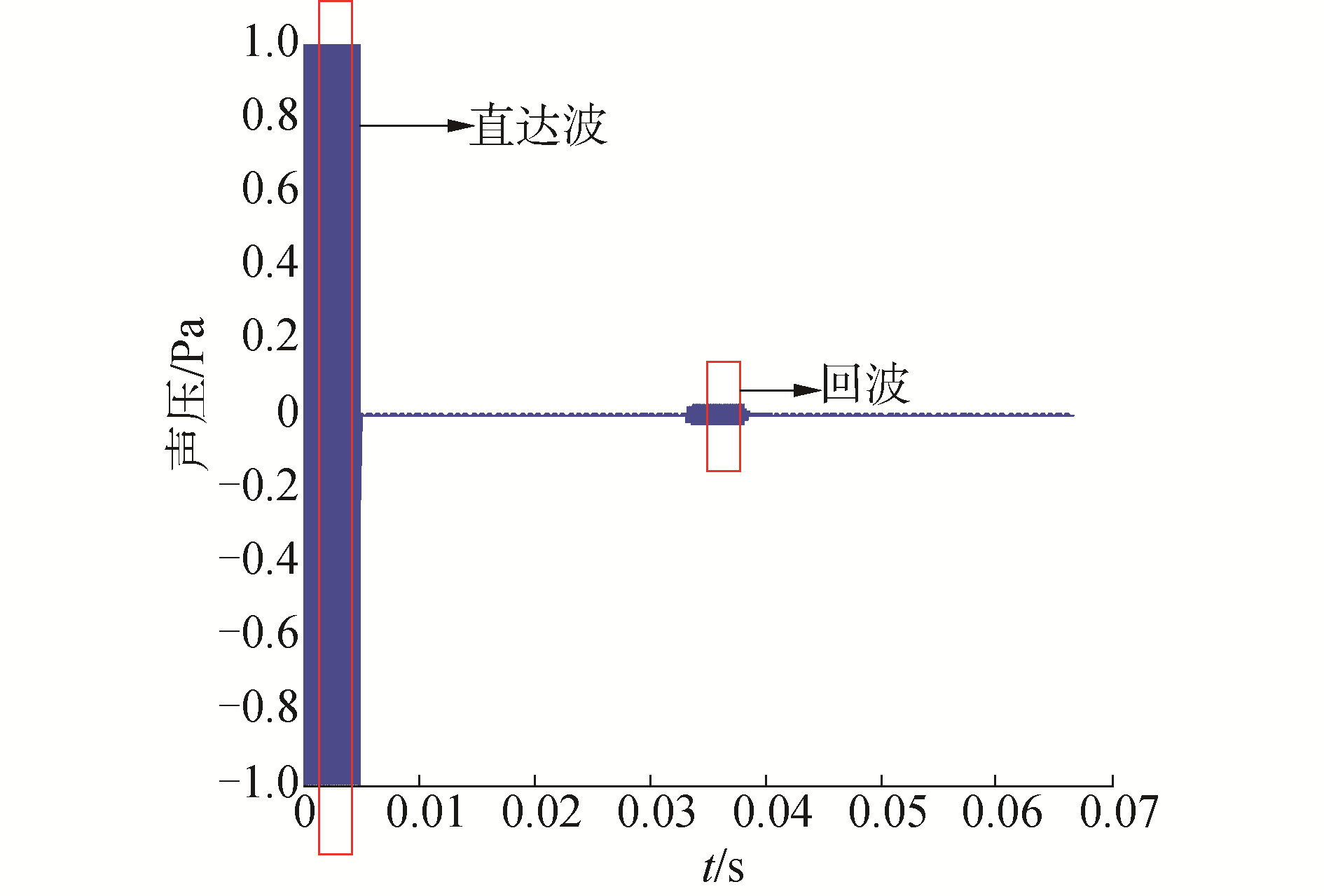Download: 图 7 理论仿真的时域回波 Fig. 7 Time-domain signal of simulation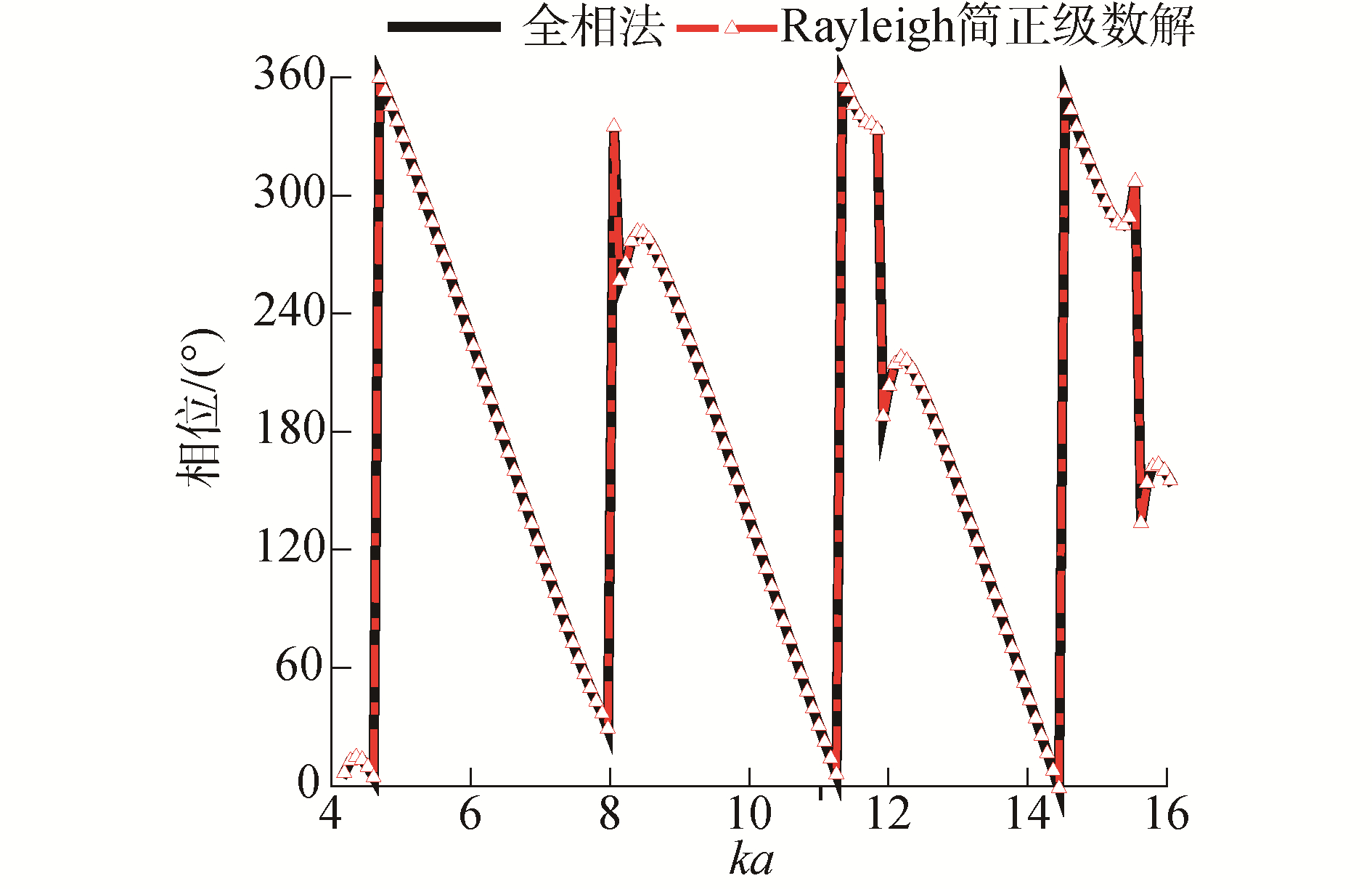Download: 图 8 回波仿真与Rayleigh简正级数解相频特性对比 Fig. 8 Frequency-response comparison between time-domain simulation and Rayleigh normal mode solution

3 水下球体目标声散射实验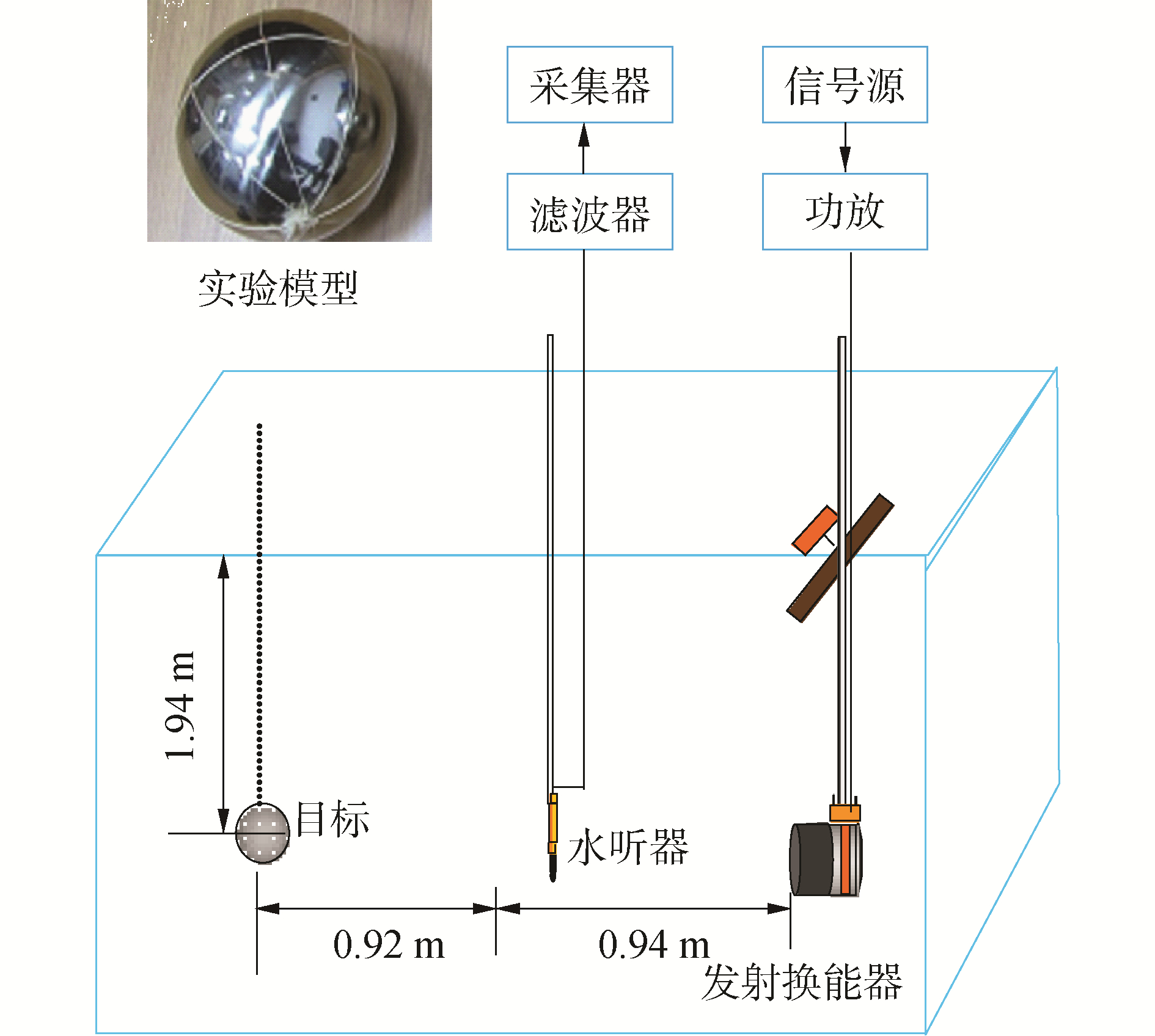Download: 图 9 实验模型和装置布放 Fig. 9 Experimental model and layout diagram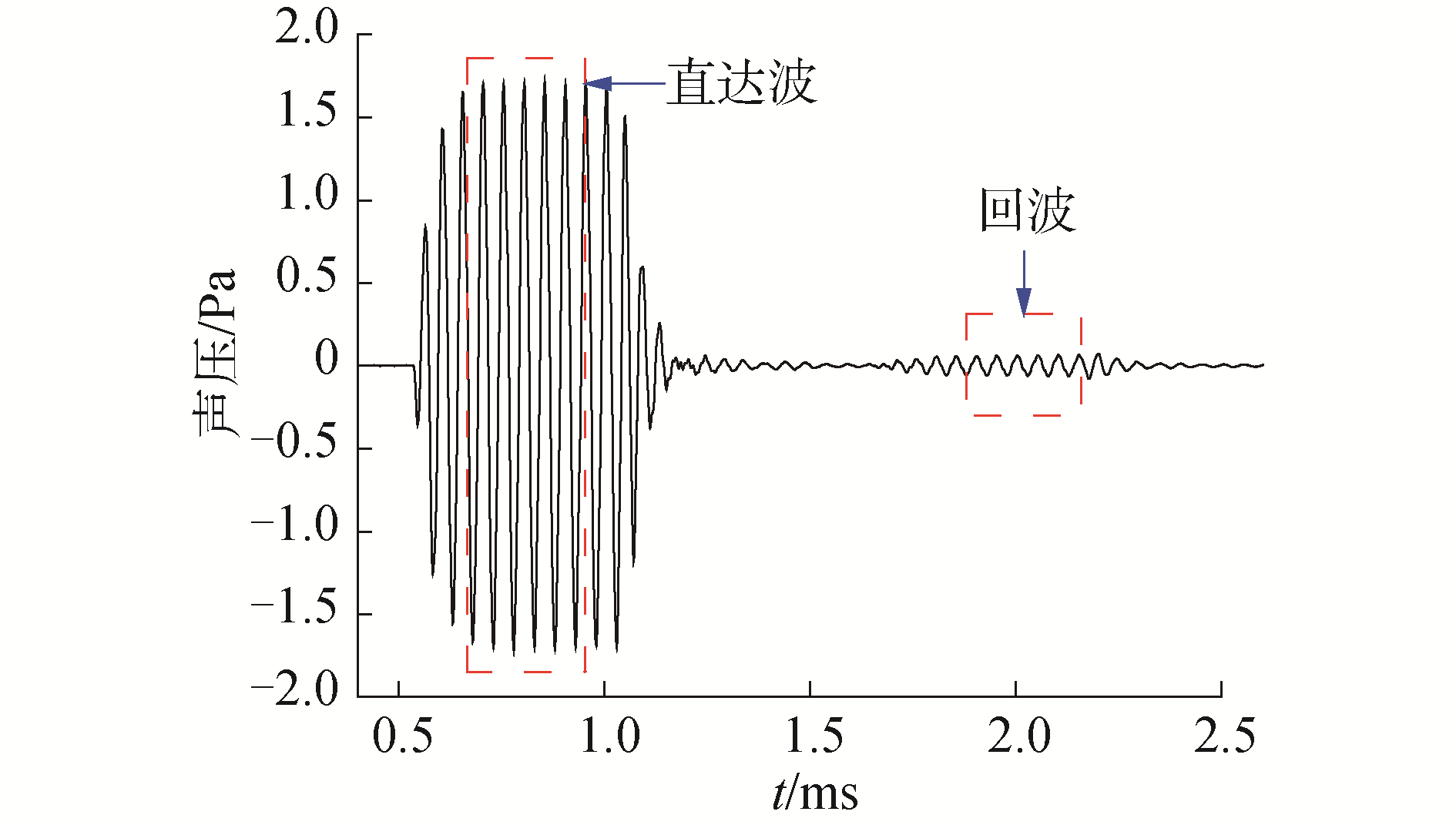Download: 图 10 时域回波信号 Fig. 10 Time-domain echo signal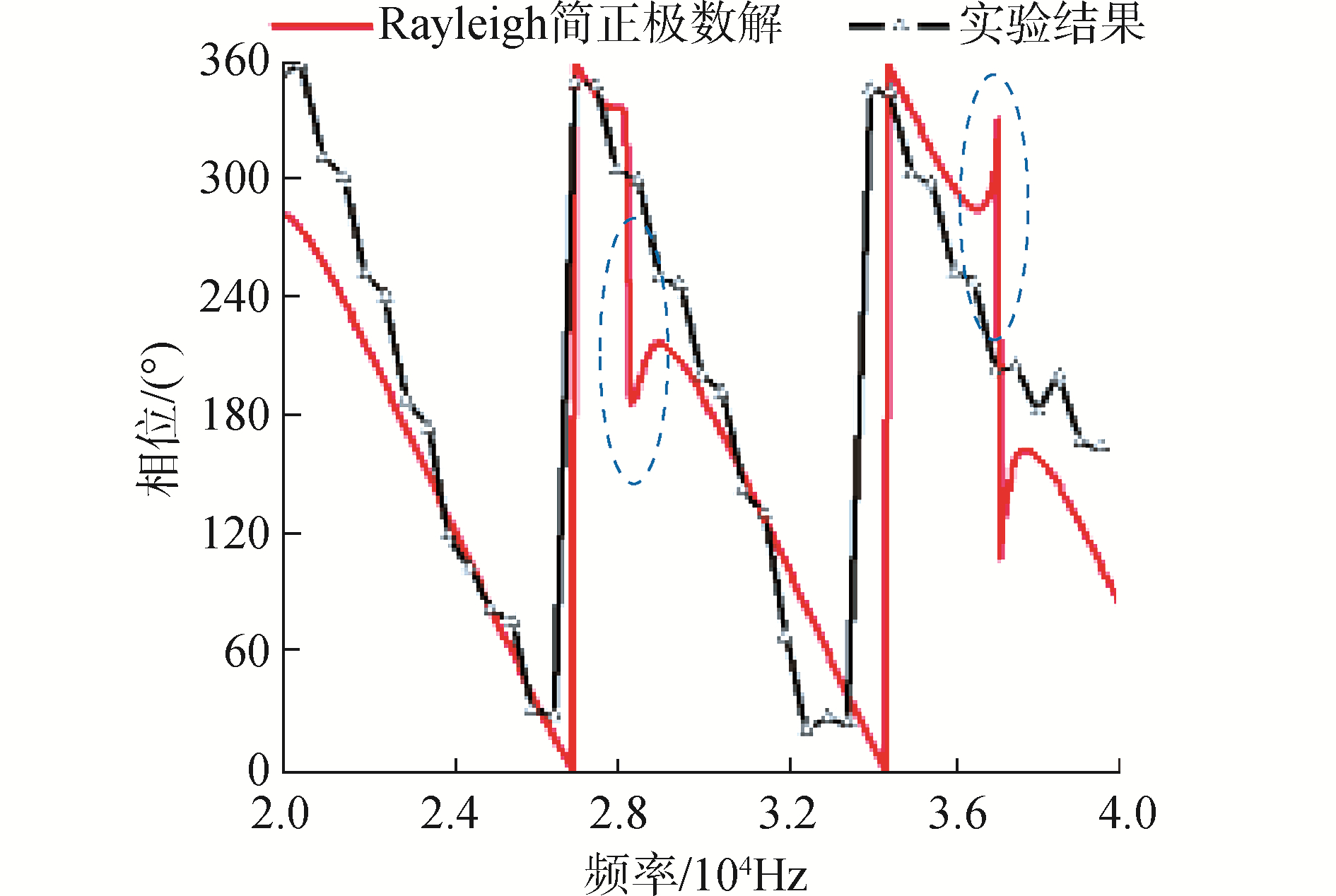Download: 图 11 实验结果与Rayleigh简正级数解对比 Fig. 11 Comparison of theoretical and experimental results

4 结论

1) 刚性球在ka>2π时相位随频率呈现接近线性变化。

2) 弹性球由于弹性波共振的出现打破了类线性变化规律，特别是在弹性波共振频率处出现相位跳变现象。

3) 不同材料、不同壳厚的弹性球壳相频特性有很大的差异，这为利用相位信息进行目标识别提供了可利用特征。

4) 通过内部真空弹性球壳的水池声散射实验，利用全相位法获取了其声散射相位特性，与理论预报结果相比吻合较好。

  ATKINS P R, COLLINS T, FOOTE K G. Transmit-signal design and processing strategies for sonar target phase measurement[J]. IEEE journal of selected topics in signal processing, 2007, 1(1): 91-104. DOI:10.1109/JSTSP.2007.897051 (0)  MITRI F G, GREENLEAF J F, FELLAH Z E A, et al. Investigating the absolute phase information in acoustic wave resonance scattering[J]. Ultrasonics, 2008, 48(3): 209-219. (0)  黄翔东, 王兆华. 全相位FFT谱与传统FFT谱对比实验研究[J]. 计算机工程与应用, 2010, 46(9S): 334-336. HUANG Xiangdong, WANG Zhaohua. Comparative experimental study between all-phase FFT and conventional FFT spectral analysis[J]. Computer engineering and applications, 2010, 46(9S): 334-336. (0)  MITRI F G. Generalized theory of resonance excitation by sound scattering from an elastic spherical shell in a nonviscous fluid[J]. IEEE transactions on ultrasonics, ferroelectrics, and frequency control, 2012, 59(8): 1781-1790. DOI:10.1109/TUFFC.2012.2382 (0)  GAUNAURD G C, WERBY M F. Sound scattering by resonantly excited, fluid-loaded, elastic spherical shells[J]. The journal of the acoustical society of America, 1991, 90(5): 2536-2550. DOI:10.1121/1.402059 (0)  范威, 郑国垠, 范军. 充水圆柱壳声散射的环绕波分析[J]. 声学学报, 2010, 35(4): 419-426. FAN Wei, ZHENG Guoyin, FAN Jun. Analysis of circumferential waves on a water-filled cylindrical shell[J]. Acta acustica, 2010, 35(4): 419-426. (0)  卢笛.基于有限元原理的弹性目标声散射计算[D].哈尔滨: 哈尔滨工程大学, 2014. LU Di. Researches on acoustic scattering of elastic target on finite element methods[D]. Harbin: Harbin Engineering University, 2014. (0)  王兆华, 黄翔东, 杨尉. 全相位FFT相位测量法[J]. 世界科技研究与发展, 2007, 29(4): 28-32, 27. WANG Zhaohua, HUANG Xiangdong, YANG Wei. The measuring phase method of All-Phase FFT[J]. World sci-tech R & D, 2007, 29(4): 28-32, 27. DOI:10.3969/j.issn.1006-6055.2007.04.006 (0)  HUANG Xiaohong, WANG Zhaohua, CHOU Guoqiang. New method of estimation of phase, amplitude, and frequency based on all phase FFT spectrum analysis[C]//Proceedings of 2007 International Symposium on Intelligent Signal Processing and Communication Systems. Xiamen, 2007: 284-287. (0)  吴国乔.全相位方法在频谱分析及数字滤波上的应用[D].天津: 天津大学, 2006. WU Guoqiao. The application of the all phase method in the spectrum analysis and digital filter[D]. Tianjin: Tianjin University, 2006. (0)  卜考英.水下目标回波特性预报模型验证研究[D].上海: 上海交通大学, 2006. BU Kaoying. Validation research on echo characteristic prediction model of underwater targets[D]. Shanghai: Shanghai Jiao Tong University, 2006. (0)